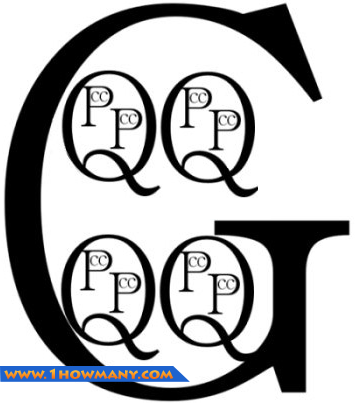﻿ How Many Pints in a Gallon
www.1howmany.com
Units of Measure / How Many Pints in a Gallon

# How Many Pints in a Gallon

Gallons1 and pints2 are common liquid measurement units though sometimes they are also used to measure dry substances. Currently they are spread mainly in the USA and Australia. If you talk about US customary gallons and pints, it is not difficult to find the right answer to the question, “How many pints are in one gallon?” It is widely known that one US gallon consists of 8 US pints.

US and Imperial Gallons and Pints

There are different definitions of the gallon and the pint, but nowadays only three of them are in use. They are the imperial gallon (about 4.54 liters), the US liquid gallon (3.78 liters), and the US dry gallon (about 4.4 liters). To say the truth, dry gallons are also used very rarely, so it makes sense to consider only US liquid gallons and imperial gallons.

As for pints, we have the same picture. There are three main definitions of the pint: the imperial pint (about 0.57 liters), the US liquid pint (about 0.47 liters), and the less common US dry pint (about 0.55 liters).

If you are going to convert gallons to pints within the scope of the same system, you will have no problems, because in all of them eight pints make up one gallon.

• One US liquid gallon equals to 8 US liquid pints
• One US dry gallon equals to 8 US dry pints
• One imperial gallon equals to 8 imperial pints

All you have to do is to multiply the number of gallons by eight, and you will know how many pints are in them.

But converting units from different systems is not so easy. We have composed the special table that will help you to do it, though you may need a calculator to perform this task.

Using Pints and Gallons

Pints and gallons are the common measurements for distributing different fluids. In the USA, petrol is usually dispensed by gallons. The pint is the traditional volume unit for selling beer in many countries. Pints and gallons are also used in cooking recipes.

The following picture and video will help you to memorize how many pints, quarts and cups are in one gallon.

• G is a gallon
• Q is a quart
• P is a pint
• C is a cup1. The gallon is a non-metric liquid measurement unit accepted in many countries. The US liquid gallon (about 3.785 liters) and the imperial gallon (about 4.54 liters) are the most common definitions of this unit. The gallon consists of eight pints.
2. The pint (abbr. p or pt) is a non-metric unit of liquid measure widely used in the USA and several other countries. The US pint is equivalent to approximately 0.47 liters.
You may be interested in: